### RN Financial Research Centre Blog Cases Courses Home

⇦ Back to Resources

# Lesson 3 - Videos

## Simple Linear Regression and Confidence Intervals

##### Slide 1:

Tibshirani: Hello, everyone. We’re going to continue now our discussion of supervised learning. Linear regression is the topic, and actually, as we’ll see, it’s a very simple method. But that’s not a bad thing. Simple’s actually good. As we’ll see, it’s very useful, and also the concepts we learned in linear regression are useful for a lot of the different topics in the course. So this is chapter three of our book. Let’s look at the first slide. As we say, linear regression is a simpler approach to supervised learning that assumes the dependence of the outcome, $$Y$$, on the predictors, $$X_1$$ through $$X_p$$, is linear. Now, let’s look at that assumption.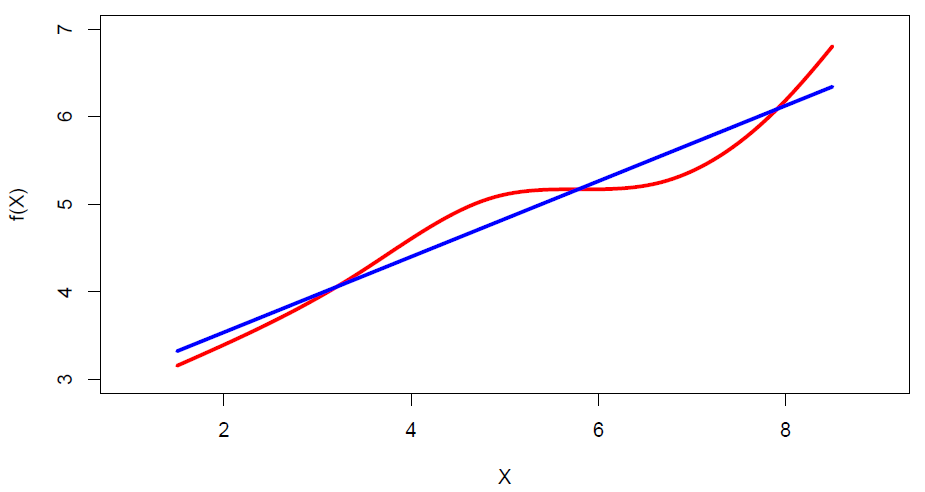Figure 3.1

So in this little cartoon example, the true regression function is red. And it’s not linear, but it’s pretty close to linear. And the approximation in blue there, the blue line, it looks like a pretty good approximation. Especially if the noise around the true red curve, as we’ll see, is substantial, the regression curve in blue could be quite a good approximation. So although this model is very simple– I think there’s been sort of a tendency of people to think simple is bad. We want to use things that are complicated and fancy and impressive. Well, actually, I want to say the opposite. Simple is actually very good. And this model being very simple, it actually works extremely well in a lot of situations. And in addition, the concepts we learn in regression are important for a lot of the other supervised learning techniques in the course. So it’s important to start slowly, to learn the concepts of the simple method, both for the method itself and for the future methods in the course. So what is the regression model?

##### Slide 2:

Tibshirani: Well, before I define the model, let’s actually look at the advertising data, which I’ve got in the next slide.

##### Slide 3:

Tibshirani: This data looks at sales as a function of three kinds of advertising, TV, radio, and newspaper. And here I’ve got scatter plots of the sales versus each of the three predictors individually.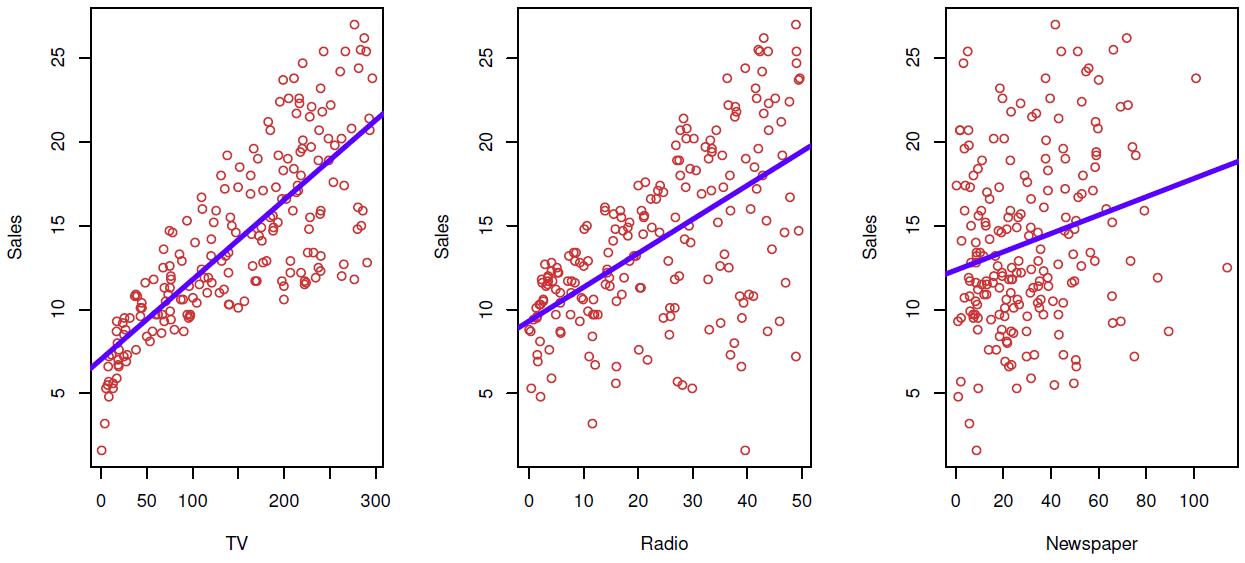Figure 3.2Linear fit of Sales vs. TV, Radio, Newspaper

And you can see the approximations by the regression line are pretty good. Looks like, for the most part, they’re reasonable approximations. On the left side, maybe for low TV advertising, the sales are actually lower than expected, which we can see here. But for the most part, the linear approximation is reasonable, partly because, again, the amount of noise around the curve, around the line, is quite large. So even the actual regression function was nonlinear, we wouldn’t be able to see it from this data. So this is an example of how it’s this crude approximation, which is potentially quite useful.

##### Back to Slide 2:

Tibshirani: So what are the questions we might ask of this kind of data, and would you might ask the regression model to help us to answer? Well, one question is, is the relationship between the budget of advertising and sales. That’s the sort of overall global question, do these predictors have anything to say about the outcome? Furthermore, how strong is that relationship? The relationship might be there, but it might be so weak as not to be useful. Now, assuming there is a relationship, which media contributed to sales? Is it TV, radio, or newspaper, or maybe all of them? If we want to use this model to predict, how well can we predict future sales? Is the relationship linear? We just discussed that already. If it’s not linear, maybe if we use a nonlinear model, we’ll be able to make better predictions. Is there synergy among the advertised media? In other words, do the media work on their own in a certain way, or do they work in combination? And we’ll talk about ways of looking at synergy later in this section.

##### Slide 4:

Tibshirani: OK, well, what is linear regression? Well, let’s start with the simplest case, where a simple model with just a single predictor. And this is the model here. It says that the outcome is just a linear function of the single predictor, $$X$$, with noise, with errors, the $$\epsilon$$.

$Y = \beta_0 + \beta_1X + \epsilon$

So this is just the equation of a line. We’ve added some noise at the end to allow the points to deviate from the line. The parameters that are the constants, $$\beta_0$$ and $$\beta_1$$ are called parameters or coefficients. They’re unknown. And we’re going to find the best values to make the line fit as well as possible. So you see a lot terminology. Those parameters are called the intercept and slope, respectively, because they’re the intercept and slope of the line. And again, we’re going to find the best-fitting values to find the line that best fits the data. And we’ll talk about that in the next slide. But suppose we have for the moment some good values for the slope and intercept. Then we can predict the future values simply by plugging them into the equation. So if we have a value of $$x$$, we want it for what you want to predict. The $$x$$ might be, for example, the advertising you budget for TV. And we have our coefficients that we’ve estimated. We simply plugged them into the equation, and our prediction for future sales at that value of $$x$$ is given by this equation.

$\hat{y} = \hat{\beta}_0 + \hat{\beta}_1x$

And you’ll see throughout the course, as is standard in statistics, we put a hat, this little symbol, over top of a parameter to indicate the estimated value which we’ve estimated from data. So that’s a sort of funny way. That’s become a standard convention.

##### Slide 5:

Tibshirani: So how do we find the best values of the parameters? Well, let’s suppose we have the prediction for a given value of the parameters at each value in the data set.

$\hat{y}_i = \hat{\beta}_0 + \hat{\beta}_1x_i$

Then the residual, what’s called the residual, is the discrepancy between the actual outcome and the predicted outcome.

$e_i = y_i - \hat{y}_i$

So we define the residual sum of squares as the total square discrepancy between the actual outcome and the fit. The equivalent, if you write that out in detail, it looks like this, right?

$RSS = e\begin{smallmatrix} 2\\ 1 \end{smallmatrix} + e\begin{smallmatrix} 2\\ 2 \end{smallmatrix} + \dots + e\begin{smallmatrix} 2\\ n \end{smallmatrix}$

This is the error, the residual for the first observation, square, second, et cetera. So it makes sense to say, well, I want to choose the values of these parameters, the intercept and slope, to make that as small as possible. In other words, I want the line to fit the points as closely as possible. Let’s see.

##### Slide 6:

Tibshirani: This next slide– I’ll come back to the equation in the previous slide, but this next slide shows in pictures.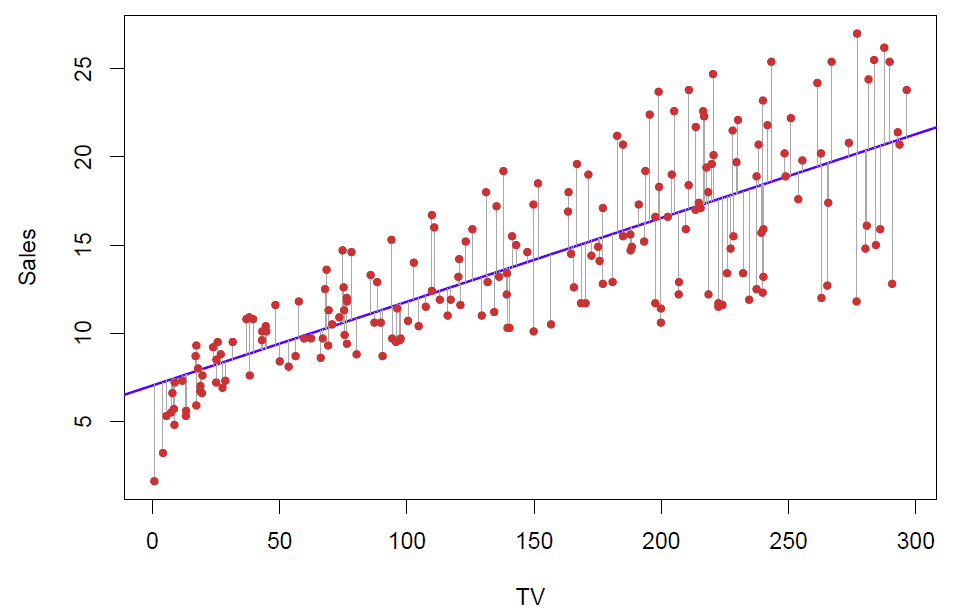Figure 3.3Residuals of the linear model

So here are the points. Each of these residuals is the distance of each point from the line. And I square up these distances. I don’t care if I’m below or above. I’m not going to give any preference. But I want the total score squared distance of all points to the line to be as small as possible. Because I want the line to be as close as possible to the points. This is called the least squares line. There’s a unique line that fits the best in this sense.

##### Back to Slide 5:

Tibshirani: And the equations for the slope-intercept are given here. Here’s the slope and the intercept.

$\hat{\beta}_1 = \frac{\sum_{i=1}^{n}(x_i - \bar{x})(y_i - \bar{y})}{\sum_{i=1}^{n}(x_i - \bar{x})^2}\text{, }\hat{\beta}_0 = \bar{y} - \hat{\beta}_1\bar{x}\\ \textit{ where }\bar{y} \equiv \frac{1}{n}\sum_{i=1}^{n}y_i\textit{ and }\bar{x} \equiv \frac{1}{n}\sum_{i=1}^{n}x_i\textit{are the sample means.}$

So just basically a formula involving the observations for the slope-intercept, and these are the least squares estimates. These are the ones that minimize the sum of squares.

##### Slide 7:

Tibshirani: Of course, a computer program like R or pretty much any other statistical program will compute that for you. You don’t need to do it by hand. OK, so we have our data for a single predictor. We’ve obtained the least squares estimates. Well, one question we want to know is how precise are those estimates. In particular, we want to know what? We want to know, for example, is the slope 0? If the slope is 0, that means there’s no relationship between $$y$$ and $$x$$. Suppose we obtained a slope of 0.5. Is that bigger than 0 or not? Well, we need a measure of precision. How close is that actually to 0? Maybe if we got a new dataset from the same population, we get a slope of minus 0.1. Then the 0.5 is not as impressive as it sounds. So we need what’s called the standard error for the slope and intercept. Well, here are the formulas for the standard errors of the slope and intercept.

$\textit{Standard error of slope, }\text{SE}(\hat{\beta}_1)^2 = \frac{\sigma^2}{\sum_{i=1}^{n}(x_i - \bar{x})^2}\\ \textit{Standard error of intercept, }\text{SE}(\hat{\beta}_0)^2 = \sigma^2[\frac{1}{n} + \frac{\bar{x}^2}{\sum_{i=1}^{n}(x_i - \bar{x})^2}]\\ \textit{where }\sigma^2 =\text{ Var}(\epsilon)$

Here’s the one we really care about. This is the square standard error of the slope. Its sigma squared, where sigma squared is the noise, the variance of the errors around the line. And this is interesting. It says this is the spread of the $$x$$’s around their mean. This actually makes sense. It says the standard error of the slope is bigger if my noise variance is bigger. That makes sense. The more noise around the line, the less precise the slope. This says the more spread out the $$x$$’s, the more precise the slope is. And that actually makes sense. I’ll go back to the sixth slide.

##### Back to Slide 6:

Tibshirani: The more spread out these points are, the more I have the slope pinned down. Think of like a teeter totter. Imagine I had the points, they were all actually concentrated around 150. Then this slope could vary a lot. I could turn it, change the slope, and still fit the points about the same. But the more the points are spread out in $$x$$ across the horizontal axis, the better pinned down I have the slope, the less slop it has to turn. So this also says you have a choice of which observations to measure. And so maybe in an experiment where you can design, you should pick your predictor values, the $$x$$’s, as spread out as possible in order to get the slopes estimated as precisely as possible.

##### Slide 7:

Tibshirani: OK. So that’s the formula for the standard error of the slope and for the intercept. And what we do with these? Well, one thing we can do is form what’s called confidence intervals. So a confidence interval is defined as a range so that it has a property that with high confidence, 95%, say, which is the number that we’ll pick, that that range contains the true value with that confidence. In other words, to be specific, if you want a confidence interval of 95%, we take the estimate of our slope plus or minus twice the estimate of the standard error.

$\hat{\beta}_1 \pm 2 \cdot \text{SE}(\hat{\beta}_1)$

And this, if errors are normally distributed, which we typically assume, approximately, this will contain the true value, the true slope, with probability 0.95.

##### Slide 8:

Tibshirani: OK, so what we get from that is a confidence interval, which is a lower point and an upper point, which contains the true value with probability 0.95 under repeated sampling. Now, what does that mean? This is a little tricky to interpret that. Let’s see in a little more detail what that actually means. Let’s think of a true value of beta, $$\beta_1$$, which might be 0 in particular, which means the slope is 0. And now let’s draw a line at $$\beta_1$$. Now imagine that we draw a dataset like the one we drew, and we get a confidence interval from this formula, and that confidence interval looks like this. So this one contains a true value because they’ve got the line is in between in the bracket. Now I get a second dataset from the same population, and I form this confidence interval from that dataset. It looks a little different, but it also contains a true value. Now I get a third data set, and I do the least squares computation. I form the confidence interval. Unluckily, it doesn’t contain the true value. It’s sitting over here. It’s above beta one. Beta one’s below the whole interval. And I get another dataset. Maybe I miss it on the other side this time. And I get another dataset, and I contain the true value. So we can imagine doing this experiment many, many times, each time getting a new dataset from the population, doing least squares computation, and forming the confidence interval. And what the theory tells us is that if I form, say, 100 confidence intervals, 100 of these brackets, 95% of the time, they will contain the true value. The other 5% of the time, they will not contain the true value. So I can be pretty sure that the interval contains the true value if I form the confidence interval in this way. I can be sure at probability 0.95. So for the advertising data, the confidence interval for beta one is 0.042 to 0.053. This is for TV sales. So this tells me that the true slope for TV advertising is– first of all, it’s greater than 0. In other words, having TV advertising does have a positive effect on sales, as one would expect. OK, so that completes our discussion of standard errors and confidence intervals. In the next segment, we’ll talk about hypothesis testing, which is a closely related idea to confidence intervals.

## Hypothesis Testing

##### Slide 9:

Tibshirani: Welcome back. We just finished talking about confidence intervals in the previous segment, and now we’ll talk about hypothesis testing, which is a closely related idea. We want to ask a question about a specific value of a parameter, like is that coefficient 0? In statistics, that’s known as hypothesis testing. So hypothesis testing is a test of a relationship between– it’s a test of a certain value of a parameter. In particular, here the hypothesis test we’ll make is that, is that parameter 0? Is the slope 0? So what’s called the null hypothesis is that there’s no relationship between $$X$$ and $$Y$$. In other words, $$\beta_1 = 0$$. That’s the equivalent statement. The alternative hypothesis is that there is some relationship between $$X$$ and $$Y$$. In other words, $$\beta_1 \neq 0$$. And $$\beta_1$$ could be positive or negative. So mathematically, this corresponds to $$\beta_1$$ being 0. Is the null hypothesis $$\beta_1$$ not equal 0? So that’s often the question you want to ask. That’s usually the first question you want to ask about the predictors.

##### Slide 10:

Tibshirani: So to test the null hypothesis, we form what’s called a t-statistic. We take the estimated slope divided by the standard error. This will approximately have a t-distribution with $$n - 2$$ degrees of freedom assuming that the null hypothesis is true. Now, what is a t-distribution? You don’t have to worry too much about that. It’s basically you look this up in a table or, nowadays software will compute it for you. It’s basically a normal random variable except for small numbers of samples. $$n$$ is a little bit different. In any case, you ask the computer to compute the p-value based on this statistic. p-value is the probability of getting the value of $$t$$ at least as large as you got in absolute value.

##### Slide 11:

Tibshirani: So for the advertising data using, again, just TV, here are the results.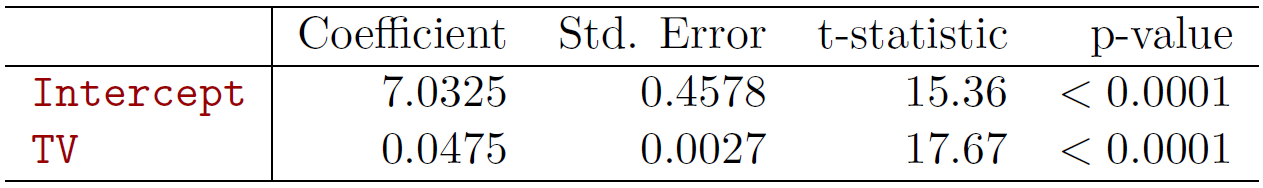Figure 3.4

Here are the slope and intercept of that line. So saw the least squares line. Standard errors. Here are the t-statistics. That’s just the coefficient divided by the standard error. The one we care most about is for TV. The intercept isn’t really very interesting. That’s telling us what happens– what are the sales when the TV is 0? TV’s budget is 0. But the one we care most about here is this guy. So this is measuring the effect of TV advertising on sales. And the t-statistic is huge. It turns out in order to have a p-value of below 0.05, which is quite significant, you need a t-statistic of about 2. We’re at 17, so it’s very, very significant. So the p-value is very, very small. So how do we interpret this? It says the chance of seeing this data, under the assumption that the null hypothesis– so there’s no effect of TV advertising on sales– is less than 10 to the minus 4. So it’s very unlikely to have seen this data. It’s possible, but very unlikely under the assumption that TV advertising has no effect. Our conclusion, therefore, is that TV advertising has an effect on sales– as we would hope.

##### Slide 12:

Tibshirani: OK? So we’ve seen how to fit a model with a single predictor and how to assess the slope of that predictor, both in terms of confidence intervals and hypothesis test.

##### Slide 36:

Hastie: So here’s a nice, pretty picture of the regression surface, sales as a function of TV and radio.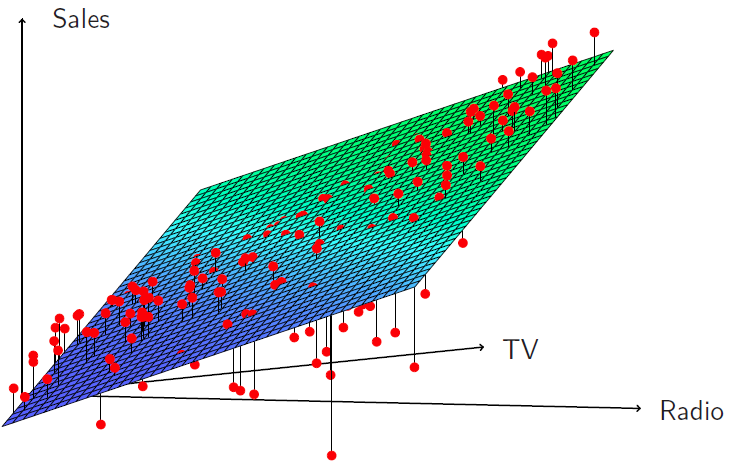Figure 3.11

And we see that when the levels of either TV or radio are low, then the true sales are lower than predicted by the linear model. But when advertising is split between the two media, then model tends to underestimate sales. And you can see that by the way the points stick out of the surface at the two ends, or below the surface in the middle.

##### Slide 37:

Hastie: So how do we deal with interactions or include them in the model? So what we do is we put in product terms. So here we have a model where we have a term for TV and the term for radio, and then we put in a term that is the product of radio and TV.

$\text{sales} = \beta_0 + \beta_1 \times \text{TV} + \beta_2 \times \text{radio} + \beta_3 \times (\text{radio} \times \text{TV}) + \epsilon$

So we literally multiply those two variables together, and call it a new variable, and put a coefficient on that variable. Now you can rewrite that model slightly, as we’ve done in the second line over here.

$\text{sales} = \beta_0 + (\beta_1 + \beta_3 \times \text{radio}) \times \text{TV} + \beta_2 \times \text{radio}+ \epsilon$

And we’ve just collected terms slightly differently. And the way we’ve written it here is showing that by putting in this interaction, we can interpret it as the coefficient of TV, which had been originally $$\beta_1$$, is now modified as a function of radio. So as the values of radio changes, the coefficient of TV changes by amount $$\beta_3$$ times radio. So that’s a nice way of interpreting what this interaction is doing.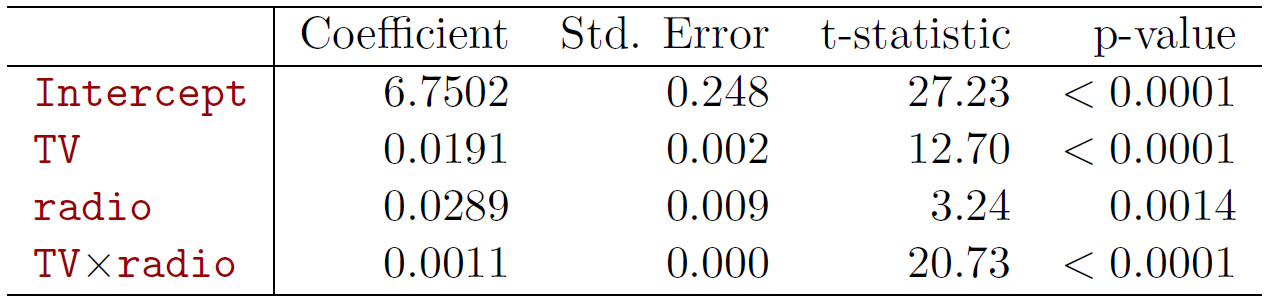Figure 3.12

And if you look at a summary of the linear model below, indeed we see that the interaction is significant, which we might have guessed from the previous picture. So in this case, the interaction really is significant.

##### Slide 38:

Hastie: So the results in this table suggest that interactions are important. The p-value for the interaction is extremely low, so there’s strong evidence in favor of the alternative here, that beta three, which was the coefficient for interaction, is not 0. We can also look at the $$R^2$$ for the model with interaction, and it’s jumped up to 96.8% compared to 89.7% by just adding this one extra parameter to the model. And we get that by adding an interaction between TV and radio.

##### Slide 39:

Hastie: Another way of interpreting this is that we have 69% of the variability in sales that remains off to fit in that it’s a model has it been explained by the interaction to because we went from 89.7 to 96.8 and if we think of that in terms of the fraction of unexplained variance, that 69% of unexplained variance.

$\frac{96.8 - 89.7}{100 - 89.7} = 69\%$

The coefficient estimates in the table suggest that an increase in TV advertising of $1,000 is associated with an increased sales of– and we plug in the numbers for $$\beta_1$$ and $$\beta_3$$. It’s 19 plus 1.1 times radio units. $(\hat{\beta}_1 + \hat{\beta}_3 \times \text{radio}) \times 1000 = 19 + 1.1 \times \text{radio units}$ Alternatively, an increase in radio advertising of$1,000 will be associated with an increase in sales of– so now we’ve written it the other way around. We factored it the other way around, and now thinking of the coefficient of radio as changing as a function of TV, and it’ll be 29 plus 1.1 times TV units.

$(\hat{\beta}_1 + \hat{\beta}_3 \times \text{TV}) \times 1000 = 29 + 1.1 \times \text{TV units}$

So you can make either of those interpretations when you put an interaction in the model.

##### Slide 40:

Hastie: Sometimes it’s the case that an interaction term has a very small p-value, but the associated main effects– in this case, TV and radio– do not. But when we put an interaction in, we tend to leave in the main effects, and we call this the hierarchy principle. And so there it’s stated if we put in an interaction, we put in the main effects, even if the p-values associated with the coefficients are not significant.

##### Slide 41:

Hastie: So why do we do this? It’s just that interactions are hard to interpret in a model without main effects– their mean: actually changes, and so it’s just generally not a good practice. Another way of saying this is that the interaction term also contains main effects, even if you fit the model with no main effect terms. So it just becomes more cumbersome to interpret.

##### Slide 42:

Hastie: Now what if we want to put in the interactions between a qualitative and a quantitative variable? Turns out thats actually easier to interpret, and we’ll see that now. So let’s look at the credit card data set again, and let’s suppose we’re going to predict balance, as before, and we’re going to use income, which is a quantitative variable, and student status, which is qualitative. And so we’ll have a dummy variable for student, which will be 1 if the person’s a student, otherwise a 0. So without an interaction, the model looks like this.

$\text{balance}_i \approx \beta_0 + \beta_1 \times \text{income}_i + \begin{cases}\beta_2 & \quad \text{if } ith \text{ person is a student}\\ 0 & \quad \text{if } ith \text{ person is not a student}\\ \end{cases}$

And we see we’ve got an intercept, we’ve got a coefficient for income, and then we’re going to have $$\beta_2$$ is the person is a student, and 0 if the person’s not a student. And another way to write that is a coefficient on income, and we just lump together the intercept and the dummy variable for student.

$\text{balance}_i = \beta_1 \times \text{income}_i + \begin{cases} \beta_0 + \beta_2 & \quad \text{if } ith \text{ person is a student}\\ \beta_0 & \quad \text{if } ith \text{ person is not a student}\\ \end{cases}$

And by grouping them like that, we can think of this as having a common slope in income, but a different intercept depending on whether the person is a student or not. And if a person as a student, the intercept is $$\beta_0 + \beta_2$$, and if the person’s not a student, it’s just $$\beta_0$$. So that’s without an interaction.

##### Slide 43:

Hastie: With interactions in the model, it takes the following form,

$\text{balance}_i \approx \beta_0 + \beta_1 \times \text{income}_i + \begin{cases} \beta_2 + \beta_3 \times \text{income}_i & \quad \text{if student}\\ 0 & \quad \text{if not student}\\ \end{cases}$

but before we study this, let’s just look at a picture of these two situations, because that’ll make things clear.

##### Slide 44: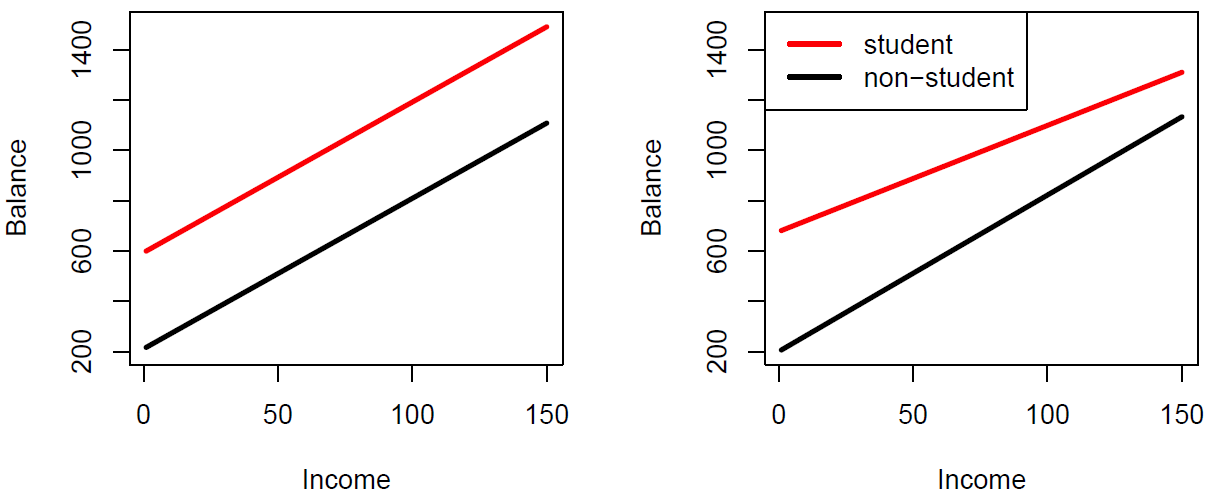Figure 3.13

Hastie: So in the left panel, we’ve got no interaction, and we see very clearly that there is a common slope for whether you’re a student or not, but just the intercept changes. But if you put an interaction between the slop of income and student status, you’re going to get both a different industry and the different slope. And so that makes it really simple explanation in this case.

##### Back to Slide 43:

Hastie: And if we look at the actual model, it looks like this. So we can write it in several different ways. And so this second term over here is showing us what happens with the interaction. And so, if you’re a student, you get both a different intercept– that’s $$\beta_2$$– and you get a different slope on income– which is $$\beta_3$$. And if you’re not a student, there’s 0, which means you get the baseline intercept and slope. And you can rearrange those terms in the following fashion and it’s telling you the same thing.

$\text{balance}_i = \begin{cases} (\beta_0 + \beta_2) + (\beta_1 + \beta_3) \times \text{income}_i & \quad \text{if student}\\ \beta_0 + \beta_1 \times \text{income}_i & \quad \text{if not student}\\ \end{cases}$

So the interpretation of interactions with categorical variables and the associated dummy variables is more simple than even in the quantitative case.

##### Slide 45:

Hastie: The other modification of the linear model is what if we want to include nonlinear effects? So here we’ve got the plot of two of the variables in the auto data set.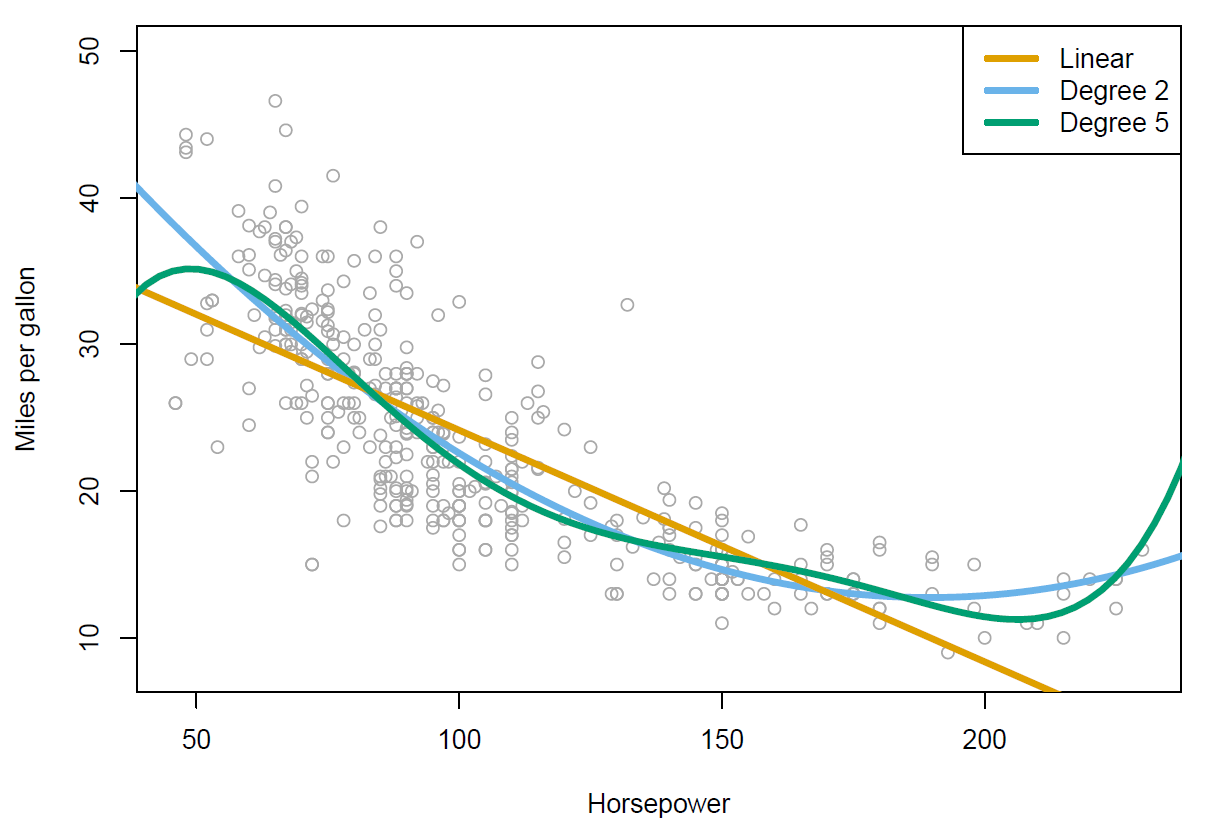Figure 3.14

So we’ve got miles per gallon against horsepower, and we’ve included three fitted models here. We’ve got the linear regression model, which is the orange curve over here. And you can see it’s not quite capturing the structure in the data. And so to improve on that, what we’ve actually done is fit two polynomial models. We’ve fit a quadratic model, which is the blue curve, and you can see that beta captures the curvature in the data than the linear model. And then we’ve also fitted a degree five polynomial, and that one looks rather wiggly. So we have an ability to fit models of different complexity, in this case, using polynomials.

##### Slide 46:

Hastie: And these are very easy to do. So just like we created an artificial dummy variable for categorical variables, we can make extra variables to accommodate polynomials. So we make a variable horsepower squared, which we just include in our data set, and now we fit a linear model with the coefficient for horsepower, and a coefficient for horsepower squared. And of course that’s a polynomial expression, and we’ll notice that that improves the fit.

$\text{mpg} = \beta_0 + \beta_1 \times \text{horsepower} + \beta_2 \times \text{horsepower}^2 + \epsilon$

We do the summary, and we see that the coefficient of both horsepower and horsepower squared are strongly significant.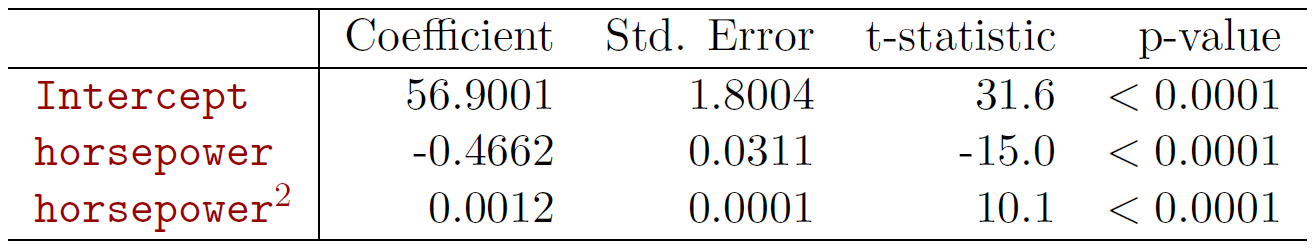Figure 3.15

And so you can do this, you can add a cubic term as well, and in the previous example, we went all the way up to a polynomial of degree five. So that’s a very easy way of allowing for nonlinearities in a variable, and still use linear regression. We still call it a linear model, because it’s actually linear in the coefficients. But as a function of the variables, it’s become nonlinear. But the same technology can be used to fit such models. So that expands the scope of linear regression enormously.

##### Slide 47:

Hastie: OK so we’ve reached the end of the session. If you’re reading along in the chapter, you’ll see there’s some topics we didn’t cover. We didn’t cover outliers. There’s non-constant variance of the error terms. High leverage points, which means if you’ve got points of observations in $$x$$ that really stick out far from the rest of the crowd, what effect they have on the model. And colinearity, if you have variables that are very correlated with each other, what happens if you include them in the model. So we’re not going to cover those here, but they’re covered in some detail in the book, and if you look at section 3.33, you’ll find coverage on that.

##### Slide 48 :

Hastie: OK, so that finishes our coverage of linear models. There are a lot of generalizations of linear model, and as I’ve hinted at already, you’ll see it’s actually quite a powerful framework. So we used similar technology for classification problems, and that will be discussed in next. So we’ll be doing logistic regression and support vector machines, which also have linear models underneath the hood, but expand the scope greatly of linear models. And then we’ll cover non linearity. So we’ll talk about techniques like kernel smoothing, and splines, and generalized additive models, some of which are also just extensions of linear models, and some of which are richer form of modelling that are for non-linearities in a more flexible way. We covered some simple interactions in linear models here, but we’ll talk about much more general techniques for dealing with interactions in a much more systematic way. And so there we’ll cover tree-based methods, and then some of the state-of-the-art techniques, such as bagging, random forests, and boosting, and these also captured non-linearities. And these really bring our bag of tools up to what we call state-of-the-art. And then another important class of methods we will discuss use what’s known as regularized fitting. And so these include ridge regression and lasso. And these have become very popular lately, especially when we have data sets where we have very large numbers of variables– so-called wide data sets, and even linear models are too rich for them, and so we need to use methods to control the variability. And so that’s all still to come, and so we have lots of nice things to look forward to.